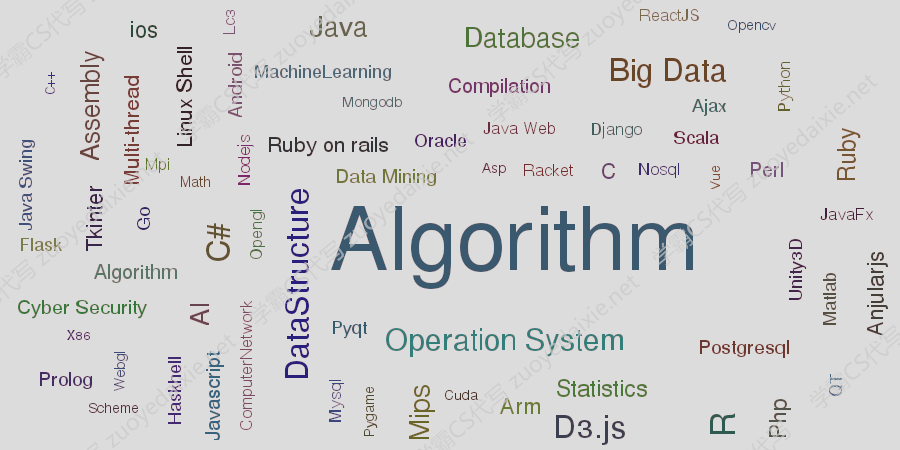# Algorithm – CSI 3131

### CSI 3131

Algorithm – 这个题目属于一个Algorithm的代写任务, 是比较有代表性的Algorithm等代写方向Midterm Sample

Question 1

Answer the following Multiple choice questions:

a. ____ scheduling is approximated by predicting the next CPU burst with an exponential average of the measured lengths of previous CPU bursts.

A) Multilevel queue B) RR C) FCFS D) SJF

b. The ____ scheduling Algorithm is designed especially for time-sharing systems.

A) SJF B) FCFS C) RR D) Multilevel queue

c. How many processes are created at the end of the following for loop?

for(i=0; i< 3 ; i++) fork();.

A) 3 B) 7 C) 8 D) 9

Question 2:

What effect does the size of the time quantum have on the performance of an RR algorithm?

Answer: At one extreme, if the time quantum is extremely large, the RR policy is the same as the FCFS policy. If the time quantum is extremely small, the RR approach is called processor sharing and creates the appearance that each of n processes has its own processor running at 1/n the speed of the real processor.

Question 3

The processes are assumed to have arrived in the order P 1 , P 2 , P 3 , P 4 , P 5 , all at time 0.

``````Process Burst Time Priority
P 1 2 2
P 2 1 1
P 3 8 4
P 4 4 2
P 5 5 3
``````

a. Draw four Gantt charts that illustrate the execution of these processes using the following scheduling algorithms: FCFS, SJF, nonpreemptive priority (a larger priority number implies a higher priority), and RR (quantum = 2).

b. What is the turnaround time of each process for each of the scheduling algorithms in part a?

c. What is the waiting time of each process for each of these scheduling algorithms?

d. Which of the algorithms results in the minimum average waiting time (over all processes)?